## I1.07: Section 5 Part 1

### Section 5: Using Models.xls to find a quadratic model

If we wish to find a model for the data on the right, this quick scatter plot of the data shows that a straight line will not be sufficient. Such parabolic shapes (similar to the path of a thrown ball) is instead represented mathematically by a quadratic formula, in which the term that contains the input variable x is squared.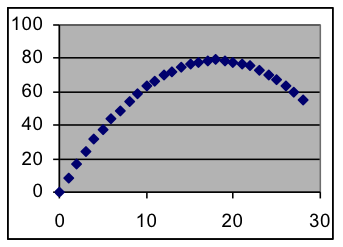There are several ways to write a quadratic formula, all of which can make the same curves. For use in fitting data, the best kind of quadratic formula is y = a (x h) 2 + v, where the parameters h and v are the x and y coordinates of the vertex of the parabola (that is, its highest or lowest point), and a is a “shape” parameter that determines how sharply (and in which direction) the parabola bends.

The quadratic formula pattern that is most convenient for fitting models: $y=a\cdot{{(x-h)}^{2}}+v$

a is a “shape” parameter controlling how much (and in which direction) the parabola bends

h is the x coordinate of the vertex (its horizontal distance from the origin)

v is the y coordinate of the vertex (its vertical distance from the origin)

Examples: $y=3\cdot{{(x-2)}^{2}}+8$   $y=2.5\cdot{{(x-7)}^{2}}-33.4$   $y=-2\cdot{{(x+3.5)}^{2}}+37$

 time height x y 0 0.0 1 8.6 2 16.8 3 24.4 4 31.5 5 37.4 6 43.8 7 48.9 8 54.4 9 58.9 10 63.3 11 66.5 12 69.7 13 72.2 14 74.5 15 76.2 16 77.8 17 78.1 18 79.0 19 78.5 20 78.0 21 76.9 22 75.6 23 73.0 24 70.3 25 67.3 26 63.7 27 60.0 28 55.0
Examples of graphs of various quadratic formulas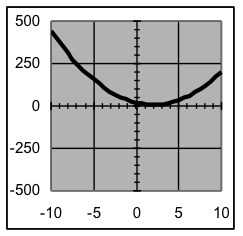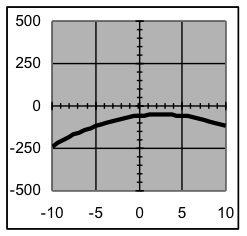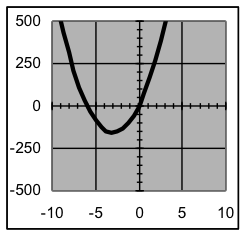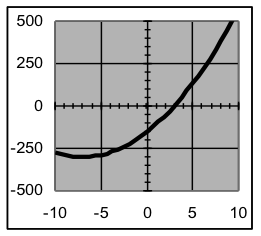$y=3\cdot{{(x-2)}^{2}}+8$ $y=-1.2\cdot{{(x-2.5)}^{2}}-50$ $y=18\cdot{{(x+3)}^{2}}-158$ $y=3\cdot{{(x+7)}^{2}}-300$

Example 5: For each of the formulas above, state the location of the vertex of the parabola formed.

Solution: Since the vertex is at (h,v) when a formula is expressed in the form,      the coordinates for the vertices are: (2, 8) (2.5, −50) (−3, −158) (−7, −300)

Note that the sign of the x vertex coordinate is the opposite of the sign that the same number has in the formula, since the h value is subtracted when forming the formula.

Quadratic models are somewhat more complicated than linear ones, as is indicated by the fact that a quadratic model has three parameters instead of two. But there is really very little difference in the fitting process from what is done for straight lines:  put the data in the appropriate worksheet,  spread the C3:E3 formulas down beside the data,  make a graph and adjust the vertex (instead of the intercept) and the shape (instead of the slope) until the model and the data match, and  write down the formula or use it to predict any values you have been asked for.